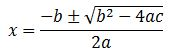# The Quadratic Formula: Definition & Example

An error occurred trying to load this video.

Try refreshing the page, or contact customer support.

Coming up next:

### You're on a roll. Keep up the good work!

Replay
Your next lesson will play in 10 seconds
• 0:02 The Quadratic Formula
• 0:39 Standard Form
• 1:03 Plugging In Your Values
• 4:10 Lesson Summary

Want to watch this again later?

Timeline
Autoplay
Autoplay
Speed

#### Recommended Lessons and Courses for You

Lesson Transcript
Instructor: Yuanxin (Amy) Yang Alcocer

Amy has a master's degree in secondary education and has taught math at a public charter high school.

Watch this video lesson to learn how you can use the quadratic formula to solve certain types of problems. Learn what kinds of equations you can solve using this formula as well as how easy it is to use this formula.

## The Quadratic Formula

The quadratic formula (x = (-b +/- sqrt (b^2 - 4ac)) / (2a)) is a famous formula that allows you to solve any type of quadratic equation. A quadratic equation is a second degree polynomial, which means that its highest exponent is 2. An example of a quadratic equation is x^2 + 3x + 4 = 0. You can see that the highest exponent is 2. The usefulness of the quadratic formula is seen when we have a quadratic equation that can't be solved by other methods. When other methods fail, the quadratic formula succeeds. Do your best to remember this formula.## Standard Form

In order to use the quadratic formula, the quadratic equation must be in standard form. What does this mean? It means that your equation must be written in decreasing order, starting with the term with the largest exponent and then going down based on the exponent. So, your quadratic equation written in standard form will look like this: ax^2 + bx + c = 0 where a, b and c are your values.

## Plugging in Your Values

Do you see how these letters are also used in the quadratic formula? Yes, when you write your quadratic equation in standard form, you can easily spot which value is your a value, your b value and your c value. Once you have all three of these values labeled, you can plug these values into your quadratic formula to solve your equation. If you see a missing term, then the letter for that term will be 0.

Let's try an example. Say we have the quadratic 2x^2 + 3x + 1 = 0 that we want to solve. We see that the equation is already written in standard form, so we can go ahead and label our a, b and c. We compare this to the standard form and we find that our a value is 2, our b value is 3 and our c value is 1. Plugging these into our quadratic formula gives us x = (-3 +/- sqrt (3^2 - (4 * 2 * 1))) / (2 * 2).

Once we have plugged in our values, we can now evaluate our formula to find our answer. The formula doesn't look nearly as bad now, does it? With all these numbers in place, it now looks manageable. Let's go ahead and evaluate to see what we get. We first tackle the inside part of the square root. We square the three to get 9. Then we multiply 4 * 2 * 1 = 8. Now we subtract the 8 from the 9 to get 1. Now we deal with the denominator by multiplying 2 * 2 = 4.

To unlock this lesson you must be a Study.com Member.

### Register to view this lesson

Are you a student or a teacher?

### Unlock Your Education

#### See for yourself why 30 million people use Study.com

##### Become a Study.com member and start learning now.
Back
What teachers are saying about Study.com

### Earning College Credit

Did you know… We have over 160 college courses that prepare you to earn credit by exam that is accepted by over 1,500 colleges and universities. You can test out of the first two years of college and save thousands off your degree. Anyone can earn credit-by-exam regardless of age or education level.